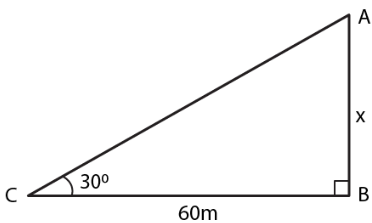Guru

# A river is 60 m wide. A tree of unknown height is on one bank. The angle of elevation of the top of the tree from the point exactly opposite to the foot of the tree on the other bank is 300. Find the height of the tree.

• 0

sir this is the question from the book -ML aggarwal( avichal publication) class 10th , chapter20 , heights and distances

we have been that A river is 60 m wide.
A tree of unknown height is on one bank.
The angle of elevation of the top of the tree from the point exactly opposite to the foot of the tree on the other bank is 300.
now we have to Find the height of the tree.

a very important question
question no 5 , heights and distances , ICSE board

Share

1. Consider AB as the tree and BC as the width of the river

C is the point which is exactly opposite to B on the other bank and 300 is the angle of elevationTake height of the tree AB = x m

Width of the river BC = 60 m

We know that

tan θ = AB/CB

Substituting the values

tan 300 = x/60

So we get

1/√3 = x/60

By cross multiplication

x = 60/√3

Multiplying and dividing by √3

x = 60/√3 × √3/√3

x = 60√3/3 = 20√3

Substituting the value of √3

x = 20 (1.732)

x = 34.640

x = 34.64 m

Hence, the height of the tree is 34.64 m.

• 0